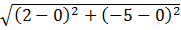### Sample Problem

What is the equation of a circle with center at the origin and a point at (2, -5)?

(A) x2+y2=25

(B) x2+y2=29

(C) x2+y2=4

(D) x2+y2=3√2

#### Solution

r =r =x2+y2=r2

x2+y2=29